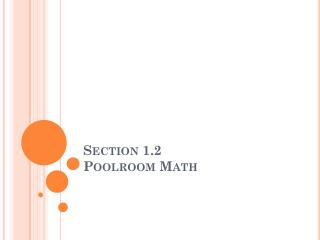Download PresentationSection 1.2 Poolroom Math# Section 1.2 Poolroom Math - PowerPoint PPT Presentation

Download Presentation##### Section 1.2 Poolroom Math

Download Policy: Content on the Website is provided to you AS IS for your information and personal use and may not be sold / licensed / shared on other websites without getting consent from its author. While downloading, if for some reason you are not able to download a presentation, the publisher may have deleted the file from their server.

- - - - - - - - - - - - - - - - - - - - - - - - - - - E N D - - - - - - - - - - - - - - - - - - - - - - - - - - -
##### Presentation Transcript

1. Section 1.2Poolroom Math

2. An Angle • An angle is formed by joining two rays together at a common endpoint. • Draw a ray on your paper. Label it ray AB. • Draw a second ray on your paper so endpoint from AB is shared by this new ray. Label the new ray AC.

3. This angle can be name in three ways.

4. The rays that make up the angle are called sides. • The common endpoint is called the vertex. sides sides vertex

5. Some angles are slightly harder to name But not A 1 has been placed in an angle. Name this angle using three letters.

6. There are three angle formed at vertex A. Name them.

7. When you draw an angle you actually draw two different angles: The angle and its reflex angle. Measures between 180o and 360o Reflex Angle Angle Measures between 0o and 180o

8. Read the measure of the angle using the other ray. Line up the zero mark of the protractor with a side of the angle. Place the center mark of the protractor at the vertex. Be sure to read the correct scale. Was your angle acute or obtuse? Smartboard File

9. Practice Measuring Angles

10. Note the congruent angle symbol indicates that two angles have the same measure.

11. A ray is an angle bisector if it divides an angle into two congruent angles. divides angle ABC into two congruent angles.

12. Look for the angle bisectors and congruent angles in the figures below. Name each angle bisector and the angle it bisects. Name all the congruent angles in the figure. Use the congruence symbol and name the angles so there is no confusion about which angle you mean.

13. So bisects No Congruent Angles

14. Virtual Pool • When a ball hits the cushion of a pool table the incoming angle is formed. two angles are formed. • When the ball leaves the cushion a second angle is formed called the outgoing angle. • The incoming angle equals the outgoing angle.

15. Use your protractor to measure angle 1. Which is the correct outgoing angle? Which point A or B will the ball hit?

16. Which point on the cushion – W, X, or Y – should the white ball hit so that the ray of the outgoing angle passes through the center of the 8-ball?

17. Compare your results with your group members’ results. Does everyone agree?

18. How would you hit the white ball against the cushion so that the ball passes over the same spot on the way back?

19. How would you hit the ball so that it bounces off three different points on the cushions without every touching cushion ? • How would you hit the ball so that it bounces off three different points on the cushions without every touching cushion ?